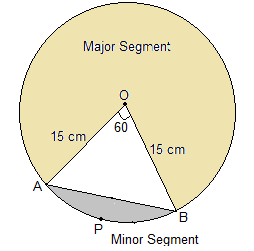# A chord of a circle of radius 15 cm subtends an angle of 60° at the centre. Find the areas of the corresponding minor and major segments of the circle. (Use π = 3.14 and √3 = 1.73)

Given

Given,

θ = 60°

Find out

The areas of the corresponding minor and major segments of the circle.

Solution∆AOB is isosceles

∴∠A = ∠B

=∠A+∠B+C=180°

=2∠A=180°-60°

=∠A=120°/2

=∠A=60°

Areas of the segments

Area of minor segment =Area of sector-Area of triangle

=∅/(360°) *πr2-√3/4(side)2

=(60°)/360*3.14*15*15-1.73/4

=706.5/6-389.25/4

=117.75-97.31

=20.44cm2

Area of major segment-Area of circle-Area of minor segment

=πr2-20.44

=3.14*15*15-20.44

=686.06cm2